edHelper subscribers - Create a new printable

Sample edHelper.com - LinearEquations Worksheet

 Name _____________________________Date ___________________
Linear Equations
State the domain and range for each. Is the relation a function?
 1 {(7.6, -0.2), (8.8, -0.2), (2.3, -4.6)}
 2 {(2.4, -3.1), (4.6, -3.4), (-2.9, -1.1), (8.3, -3.1)}
 3 {(3, 4), (-3, 4), (4, -4), (-1, -2)}

Complete.
 4 f(x) = 7x3 + 13x4 - 3x2find f(-6)
 5 f(x) = -3x + 12find f(-1)
6.
f(x) =
 -8 - 9x4 + 3x

find f(-9)

Complete.
 7 f(x) = 13x2 - 15x - 14g(x) = 15x3 + 14x2 - 15x + 12find f(3) × f(10) + f(-7) × g(9)
 8 f(x) = -15x - 10g(x) = 12x + 10find f(f(7))
 9 f(x) = -10x2 + 10x + 14g(x) = 11x + 11find f(17)-g(17)

Write each equation in standard form.
10.
 -49
+
 29
y  = x

11.
y =
 -12

Graph each equation.
12.
 y = x - 8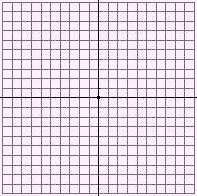13 -2y = 6 + 6xGraph each equation.
14.
x =
 35
-
 75
y15 7x + 12y = -8Write the slope of the line passing through the two points.
16.
 ( 7 , -5 ), ( 0 , 7 )

17.
 ( -5 , 2 ), ( 6 , -7 )

18.
 ( -8 , 5 ), ( 5 , -5 )

Find the intercepts of the line.
19.
 y = - x + 9

 20 3x - y = -23
 21 5x - y = -6

Graph.
22.   A line that passes through the point (-2, -6).
 slope= -123.   A line that passes through the point (-5, 0).
slope=
 35Write an equation of the line that contains the given point and has the given slope.
24.
 (6, 5), slope = -3

25.
 (0, 0), slope = -2

26.
 (-4, -5), slope = -1

Write an equation of the line that passes through the given two points.
27.
 ( -7 , 8 ), ( -4 , 11 )

28.
 ( 7 , 0 ), ( 27 , -60 )

29.
 ( 2 , 3 ), ( 8 , 0 )

Find the slope and the y-intercept of the line.
 30 5x + 3y = 25
 31 -y = 36 + 5x
 32 7x - 8y = -2

Write the slope-intercept form of the equation.
 33 5x + 12y = 13
 34 12y = -35 + 7x
 35 -y = -3 + 9x

Write an equation of the line.
36.
 y-intercept= 10 x-intercept= 2

37.
 y-intercept= -20 x-intercept= 5

State whether the lines are parallel, perpendicular, or neither.
38.
 5x - y16
=
 -378

-27y = 642 - 15x

39.   3x + 13y = 375
y =
 133
x   +  111

40.
x =
 -1711
y  +
 21911

17x + 11y = 33

Complete.
 41 Write an equation of a line that passes through the point (35, 12) and is perpendicular to the following equation:12y = 319 - 5x
42.   Write an equation of a line that passes through the point (27, 29) and is perpendicular to the following equation:
 y = 3 x - 52

 43 Write an equation of a line that passes through the point (-21, -20) and is parallel to the following equation:3x - 11y = 157

Sketch the graph of the inequality.
44.
 y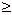x - 845.
 23
+
 13
y   >  xSketch the graph of the equation.
 46 y = |3x - 2| + 147.
y = |
 13
x| - 1Match the equation with its graph.
 2x + y = -11 5x + 6y = 28 4x - 7y = -16

 48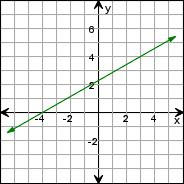49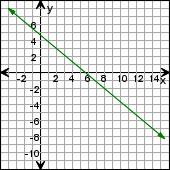50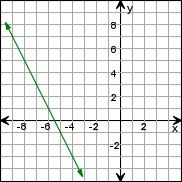Match the equation with its graph.
y =
 13
x   -
 113
 y = -4 x - 33
 y = -3 x - 30

 51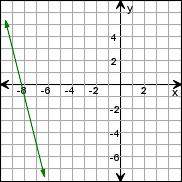52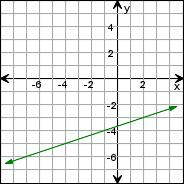53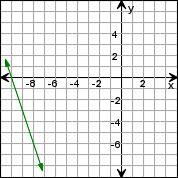Write an equation in standard form for each line.
 54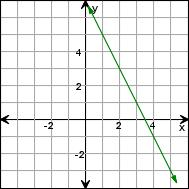55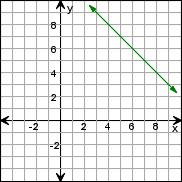56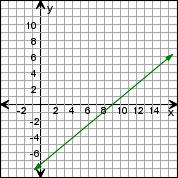Write an equation in slope-intercept form for each line.
 57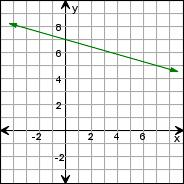58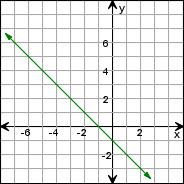59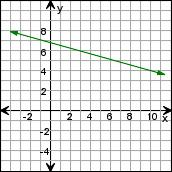Match the equation with its graph.
 4x - 5y4
=  4
 -2 - 2 y = x
 -1 - 1 y = x

 60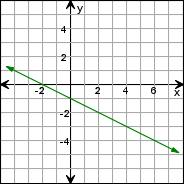61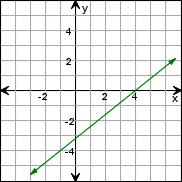62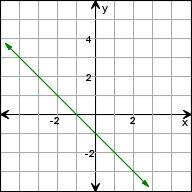Sample
This is only a sample worksheet.

edHelper subscribers - Create a new printable